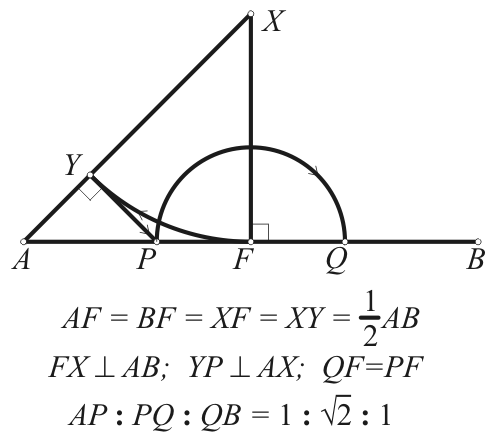Descriptive Geometry
Assignment A1 2020/21/1

A parallelepiped (in other words: a paralleogram based oblique prism) ABCDAxBxCxDx is given by the vertices A, B and D of the base ABCD, moreover the endpoint Ax of the lateral edge AAx. Construct its imeges. Divide each edge of the parallelepiped into three segments in ratio 1 : √2 : 1. The construction of dividing points can be seen in the figure below. Truncate each vertex of the parallelepiped by the plane that goes through the first dividing points of edges emanating from the vertex. Construct the images of truncated parallelepiped and show the visibility. A(70, 100, 165); B(90, 40, 195); D(130, 130, 185); Ax(40, 120, 225).